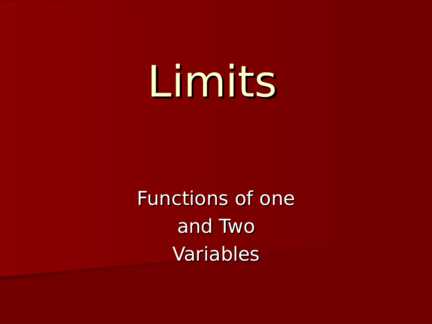# Limits for Functions of One and Two VariablesContributed by:We will be covering here:
limits of functions of one and two variables.
1. Limits
Functions of one
and Two
Variables
2. Limits for Functions of One
Variable.
What do we mean when we say that
lim f ( x) L?
x a
Informally, we might say that as x gets “closer and
closer” to a, f(x) should get “closer and closer” to L.
This informal explanation served pretty well in
beginning calculus, but in order to extend the idea to
functions of several variables, we have to be a bit more
precise.
3. Defining the Limit
Remember: the pt. lim f ( x) L
(a,f(a)) is excluded! x a
Means that
•given any tolerance T for L
L+T • we can find a tolerance t for
a
L such that
L-T •if x is between a-t and a+t,
but x is not a,
• f(x) will be between L-T and
L+T.
(Graphically, this means that the
part of the graph that lies in the
yellow vertical strip---that is,
those values that come from
a-t a a+t (a-t,a+t)--- will also lie in the
orange horizontal strip.)
4. This isn’t True for This
function!
No amount of making the
Tolerance around a smaller is
going to force the graph of
L+T that part of the function
within the bright orange
L strip!
a
lim f ( x)  L
x a
5. Changing the value of L doesn’t help
either!
L
a
In fact, there is no L that will help us. lim f ( x) does not exist.
x a
6. Functions of Two Variables
How does this extend to functions of two variables?
lim f ( x, y )  L
( x , y )  ( a ,b )
means that as (x,y) gets “closer and closer” to (a,b) , f(x,y)
gets closer and closer to L.
7. “Closer and Closer”
 The words “closer and closer” obviously have to do with
measuring distance.
 In the real numbers, one number is “close” to another if it is
within a certain tolerance---say no bigger than a+.01 and no
smaller than a-.01.
 In the plane, one point is “close” to another if it is within a
r
(x,y)
(a,b)
The vertical strip
becomes a
r
(a,b) (x,y)
9. Horizontal Strip?
L lies on the z-axis. Remember that the
We are interested function values are
in function values L+T back in the real
that lie between L numbers, so
z=L-T and z=L+T L-T “closeness” is once
again measured in
terms of
“tolerance.”
The set of all z-values
that lie between L-T
and L+T, are
“trapped” between
the two horizontal
planes z=L-T and The horizontal strip
becomes a
“sandwich”!
10. Putting it All Together
The part of the graph that
lies above the green circle
must also lie between the
two horizontal planes.
lim f ( x, y )  L
( x , y )  ( a ,b )
if given any pair of horizontal
planes about L, we can find a
circle centered at (a,b) so that the
part of the graph of f within the
cylinder is also between the
11. Defining the Limit
lim f ( x, y ) L
( x , y )  ( a ,b )
L+T
Means that
L •given any tolerance T for L